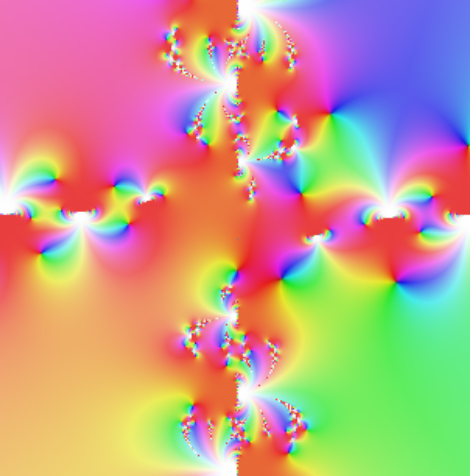# Fractal-like phase plots

Define f(z) = iz*exp(-z/sin(iz)) + 1 and g(z) = f(f(z)) + 2 for a complex argument z. Here’s what the phase plots of g look like.

The first image lets the real and imaginary parts of z range from -10 to 10.This close-up plots real parts between -1 and 0, and imaginary part between -3 and -2.The plots were produced with this Python code:

```from mpmath import cplot, sin, exp

def f(z): return 1j*z*exp(-z/sin(1j*z)) + 1

def g(z): return f(f(z)) + 2

cplot(g, [-1,0], [-3,-2], points=100000)```

The function g came from Visual Complex Functions.